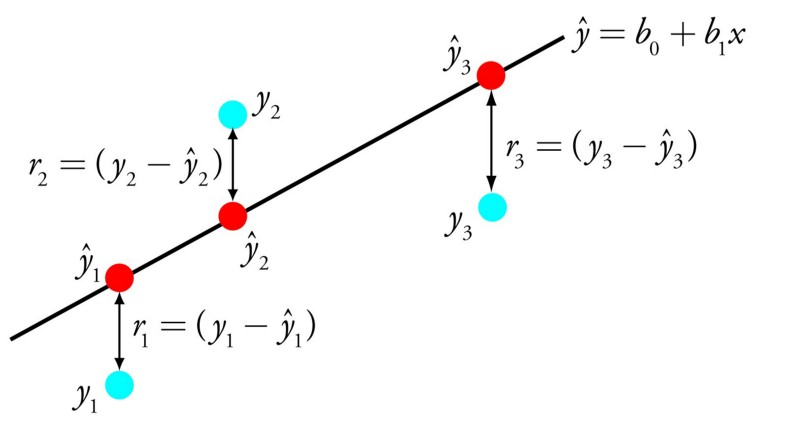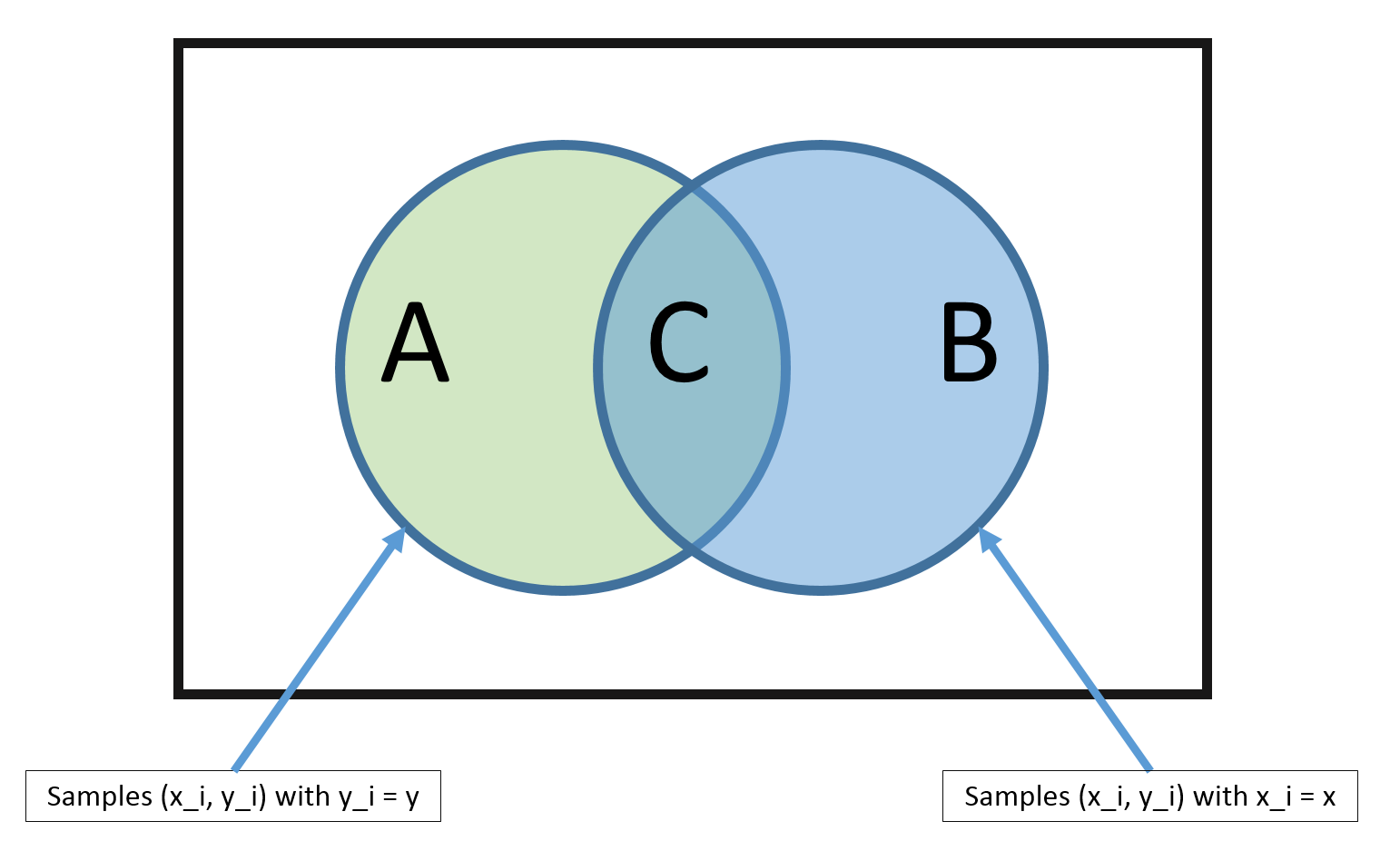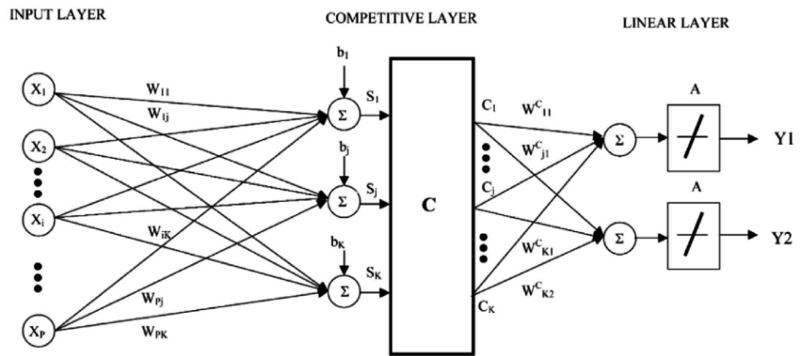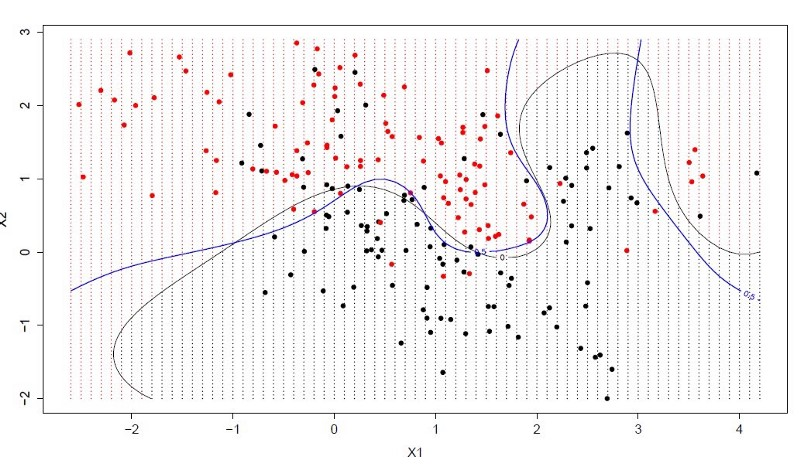{{announcement.body}}
{{announcement.title}}

# Top 10 Most Popular AI Models

DZone 's Guide to

# Top 10 Most Popular AI Models

### While AI and ML provide ample possibilities for businesses to improve their operations and maximize their revenues, there is no such thing as a “free lunch.”

· AI Zone ·
Free Resource

Comment (1)

Save
{{ articles.views | formatCount}} Views

While Artificial Intelligence and Machine Learning provide ample possibilities for businesses to improve their operations and maximize their revenues, there is no such thing as a “free lunch.”

The “no free lunch” problem is the AI/ML industry adaptation of the age-old “no one-size-fits-all” problem. The array of problems the businesses face is huge, and the variety of ML models used to solve these problems is quite wide, as some algorithms are better at dealing with certain types of problems than the others. Thus said, one needs a clear understanding of what every type of ML models is good for, and today we list 10 most popular AI algorithms:

1. Linear regression

2. Logistic regression

3. Linear discriminant analysis

4. Decision trees

5. Naive Bayes

6. K-Nearest Neighbors

7. Learning vector quantization

8. Support vector machines

9. Bagging and random forest

10. Deep neural networks

We will explain the basic features and areas of application for all these algorithms below. However, we have to explain the basic principle of Machine Learning beforehand.

All Machine Learning models aim at learning some function (f) that provides the most precise correlation between the input values (x) and output values (y). Y=f(X)

The most common case is when we have some historical data X and Y and can deploy the AI model to provide the best mapping between these values. The result cannot be 100% accurate, as otherwise, this would be a simple mathematical calculation without the need for machine learning. Instead, the f function we train can be used to predict new Y using new X, thus enabling the predictive analytics. Various ML models achieve this result by employing diverse approaches, yet the main concept above remains unchanged.

## Linear Regression

Linear regression is used in mathematical statistics for more than 200 years as of now. The point of the algorithm is finding such values of coefficients (B) that provide the most impact on the precision of the function f we are trying to train. The simplest example is
y= B0 + B1 * x,
where B0 + B1 is the function in questionBy adjusting the weight of these coefficients, the data scientists get varying outcomes of the training. The core requirements for succeeding with this algorithm is having the clear data without much noise (low-value information) in it and removing the input variables with similar values (correlated input values).

This allows using linear regression algorithm for gradient descent optimization of statistical data in financial, banking, insurance, healthcare, marketing, and other industries.

## Logistic Regression

Logistic regression is another popular AI algorithm, able to provide binary results. This means that the model can both predict the outcome and specify one of the two classes of the y value. The function is also based on changing the weights of the algorithms, but it differs due to the fact that the non-linear logic function is used to transform the outcome. This function can be represented as an S-shaped line separating the true values from false ones.The success requirements are the same as for linear regression — removing the same value input samples and reducing the quantity of noise (low-value data). This is quite a simple function that can be mastered relatively fast and is great for performing the binary classification.

## Linear Discriminant Analysis (LDA)

This is a branch of the logistic regression model that can be used when more than 2 classes can exist in the output. Statistical properties of the data, like the mean value for every class separately and the total variance summed up for all classes, are calculated in this model. The predictions allow to calculate the values for each class and determine the class with the most value. To be correct, this model requires the data to be distributed according to the Gaussian bell curve, so all the major outliers should be removed beforehand. This is a great and quite simple model for data classification and building the predictive models for it.

## Decision Trees

This is one of the oldest, most used, simplest and most efficient ML models around. It is a classic binary tree with Yes or No decision at each split until the model reaches the result node.This model is simple to learn, it doesn’t require data normalization and can help to solve multiple types of problems.

## Naive Bayes

Naive Bayes algorithm is a simple, yet very strong model for solving a wide variety of complex problems. It can calculate 2 types of probabilities:

1. A chance of each class appearing

2. A conditional probability for a standalone class, given there is an additional x modifier.The model is called naive as it operates on the assumption that all the input data values are unrelated to each other. While this cannot take place in the real world, this simple algorithm can be applied to a multitude of normalized data flows to predict results with a great degree of accuracy.

## K-Nearest Neighbors

This is quite a simple and very powerful ML model, using the whole training dataset as the representation field. The predictions of the outcome value are calculated by checking the whole data set for K data nodes with similar values (so-called neighbors) and using the Euclidian number (which can be easily calculated based on the value differences) to determine the resulting value.Such datasets can require lots of computing resources to store and process the data, suffer the accuracy loss when there are multiple attributes and have to be constantly curated. However, they work extremely fast, are very accurate and efficient at finding the needed values in large data sets.

## Learning Vector Quantization

The only major downside of KNN is the need to store and update huge datasets. Learning Vector Quantization or LVQ is the evolved KNN model, the neural network that uses the codebook vectors to define the training datasets and codify the required results. Thus said, the vectors are random at first, and the process of learning involves adjusting their values to maximize the prediction accuracy.Thus said, finding the vectors with the most similar values results in the highest degree of accuracy of predicting the value of the outcome.

## Support Vector Machines

This algorithm is one of the most widely discussed among data scientists, as it provides very powerful capabilities for data classification. The so-called hyperplane is a line that separates the data input nodes with different values, and the vectors from these points to the hyperplane can either support it (when all the data instances of the same class are on the same side of the hyperplane) or defy it (when the data point is outside the plane of its class).The best hyperplane would be the one with the largest positive vectors and separating the most of the data nodes. This is an extremely powerful classification machine that can be applied to a wide range of data normalization problems.

## Random Decision Forests or Bagging

Random decision forests are formed of decision trees, where multiple samples of data are processed by decision trees and the results are aggregated (like collecting many samples in a bag) to find the more accurate output value.Instead of finding one optimal route, multiple suboptimal routes are defined, thus making the overall result more precise. If decision trees solve the problem you are after, random forests are a tweak in the approach that provides an even better result.

## Deep Neural NetworksDNNs are among the most widely used AI and ML algorithms. There are significant improvements in deep learning-based text and speech apps, deep neural networks for machine perception and OCR, as well as using deep learning to empower reinforced learning and robotic movement, along with other miscellaneous applications of DNNs.

## Final Thoughts on 10 Most Popular AI Algorithms

As you can see, there is an ample variety of AI algorithms and ML models. Some are better suited for data classification, some excel in other areas. No model fits all sizes, so choosing the best one for your case is essential.

How to know if this model is the right one? Consider the following factors:

1. The 3 V’s of Big Data you need to process (volume, variety, and velocity of input)

2. The number of computing resources at your disposal

3. The time you can spend on data processing

4. The goal of data processing

Thus said, if some model provides 94% prediction accuracy at the cost of twice longer processing time, as compared to a 86% accurate algorithm — the variety of choices grows greatly.

However, the biggest problem usually is the general lack of high-level expertise needed to design and implement the data analysis and Machine learning solution. This is why most of the businesses choose one of Managed Services Providers specializing in Big Data and AI solutions.

Topics:
ai, ai models, algorithm, big data, deep neural networks, machine learning, ml models

Comment (1)

Save
{{ articles.views | formatCount}} Views

Opinions expressed by DZone contributors are their own.